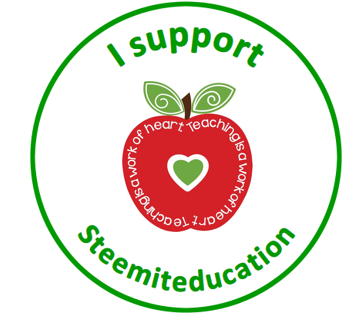# Helping learners like mathematics: worksheet for for teachers and students.

in Educationlast year

Good morning teachers and students. Welcome to my blog.
Today I will be mking attempt to share a simple worksheet on Mathematics which is meant for Primary school pupils. I do not mind sharing it with secondary students. You know why? Because I want to start from the known to unkown, so as to capture the student's interest as well as break that age-long phobia for mathematics.

### Now attempt the questions (10 marks each)

CLASS: Primary Five SUBJECT: Mathematics Duration: 2hours
Section A: Objectives (30marks)
Instruction: Choose, the correct answer, from options A – D.
Find the product of 20, 10 and 0 A. 0 B. 20 C. 200 D. 2000
There are 45 pages in a book. Each page contains 100 words. How many words has the book?
A. 4500 B. 1450 C. 450 D. 0.45
Evaluate 4×3/2 A. 12 B. 6 C. 11 D. 9
What is 1/4of a day in hours? A. 24 hours B. 6 hours C. 18 hours D. 4 hours
If your class teacher spends 2/5 of N300 to get to school, how much has he spent?
A.N600 B.N60 C.N120 D.N1,500
Evaluate 6.42 X 1000. A. 642 B. 64.2 C. 6420 D. 0.00642
What is the product of 2.36 and 0.12 A. 0.02832 B. 2.832 C. 28.32 D. 0.2832
A ribbon is 7.5cm long. Find the length of 100 pieces of such ribbons.
A. 75cm B. 7500cm C. 0.75cm D. 750cm
The following are square numbers except. A. 269 B. 169 C. 256 D. 144
Evaluate (6^2+〖12〗^2)/√81. A. 20 B. 9 C. 36 D. 180
Find the area of a square with length 13cm. A. 26cm2 B. 169cm2 C. 31cm2 D. 13cm2Thank you for reading my blog.
I am @voclab, your hive-steem tutor and strong advocate for creation of @edu-nigeria.